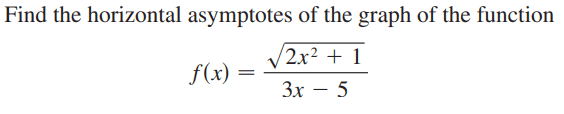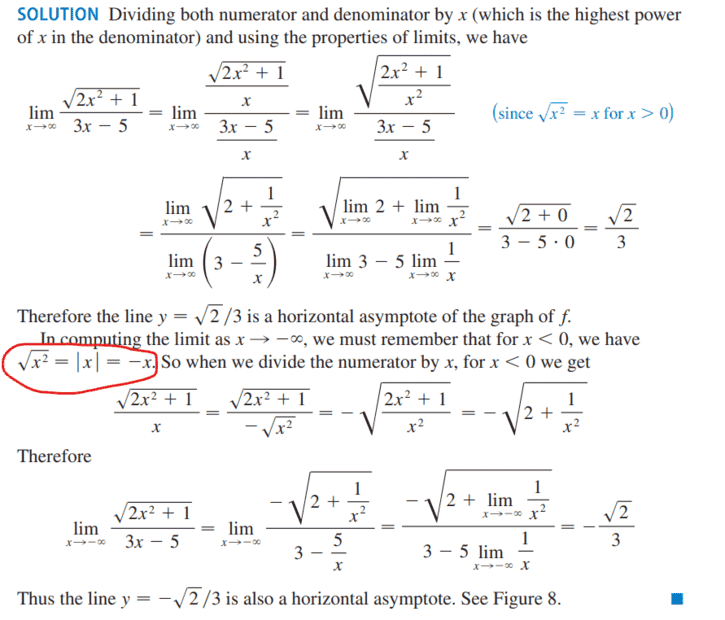# Finding the horizontal asymptote of function as x approaches negative infinity

Callumnc1
Homework Statement:
Relevant Equations:
For this problem,The solution isHowever, I don't understand the statement circled in red. I don't understand why ## x = - \sqrt{x^2}##? They did not explained why.

I remember a year ago a calculus teacher showed me how to solve this type of problem. They divided the numerator by ##\sqrt{(-x)^2} = \sqrt{(x)^2}## and the denominator by ##-x##. I don't know why you have to divide by negative x for x approaches negative infinity, but this method works and give me the same result as the books method.

Many thanks!

Homework Helper
Gold Member
Remember that this is the case where ## x\lt 0##. Therefore, ##-x## is positive and ##-x = |x| = \sqrt{x^2}##.

•Callumnc1
Callumnc1
Remember that this is the case where ## x\lt 0##. Therefore, ##-x## is positive and ##-x = |x| = \sqrt{x^2}##.

I think I understand now :)

Mentor
I remember a year ago a calculus teacher showed me how to solve this type of problem. They divided the numerator by ##\sqrt{(-x)^2} = \sqrt{(x)^2}## and the denominator by ##-x##.
You didn't say, but the teacher must have specified that x < 0. Otherwise ##\sqrt{(-x)^2} \ne -x##, so the fraction would essentially be multiplied by -1, which changes the value of the fraction.

•Callumnc1
Homework Helper
Gold Member
You didn't say, but the teacher must have specified that x < 0. Otherwise ##\sqrt{(-x)^2} \ne -x##, so the fraction would essentially be multiplied by -1, which changes the value of the fraction.
Yes. The solution included in post #1 says "we must remember that for ##x \lt 0## ...".

•Callumnc1
Mentor
Yes. The solution included in post #1 says "we must remember that for ##x \lt 0## ...".
I understand that, but I was responding to the part where the OP was remembering an event from a year ago. It wasn't stated that the teacher had specified then that x must be negative.

•Callumnc1 and FactChecker
Callumnc1
You didn't say, but the teacher must have specified that x < 0. Otherwise ##\sqrt{(-x)^2} \ne -x##, so the fraction would essentially be multiplied by -1, which changes the value of the fraction.
Thank you for your replies @Mark44 and @FactChecker !

I think my teacher said divide by numerator and denominator by (-x)^n where n is the highest degree of the denominator for taking the limit as x approaches negative infinity.

Many thanks!

Mentor
I think my teacher said divide by numerator and denominator by (-x)^n where n is the highest degree of the denominator for taking the limit as x approaches negative infinity.
That's not what my comment was about. For ##\sqrt{(-x)^2}## to be equal to -x, the teacher must have said that x < 0.

•Callumnc1
•